# ML Aggarwal Class 10 Solutions for ICSE Maths Chapter 5 Quadratic Equations in One Variable Ex 5.3

## ML Aggarwal Class 10 Solutions for ICSE Maths Chapter 5 Quadratic Equations in One Variable Ex 5.3

These Solutions are part of ML Aggarwal Class 10 Solutions for ICSE Maths. Here we have given ML Aggarwal Class 10 Solutions for ICSE Maths Chapter 5 Quadratic Equations in One Variable Ex 5.3

More Exercises

Solve the following (1 to 8) equations by using formula :

Question 1.
(i) 2x² – 7x + 6 = 0
(ii) 2x² – 6x + 3 = 0
Solution:
(i) 2x² – 7x + 6 = 0
Here a = 2, b = -7, c = 6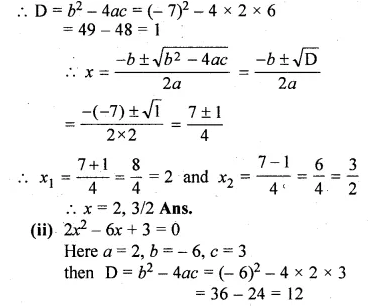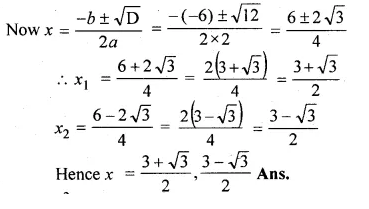Question 2.
(i) x² + 7x – 7 = 0
(ii) (2x + 3)(3x – 2) + 2 = 0
Solution:
(i) x² + 7x – 7 = 0
Here a = 1, b = 7, c = -7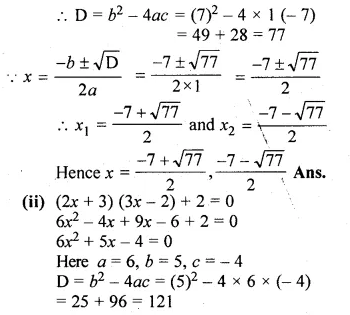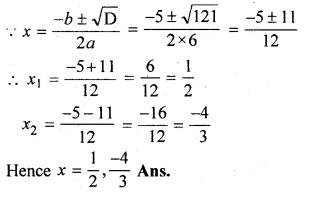Question 3.
(i)256x² – 32x + 1 = 0
(ii) 25x² + 30x + 7 = 0
Solution:
(i) 256x² – 32x + 1 = 0
Here a = 256, b = -32, c = 1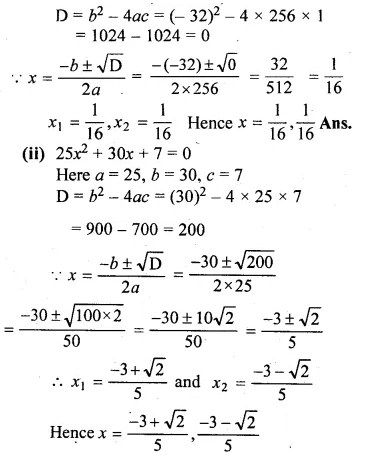Question 4.
(i) 2x² + √5x – 5 = 0
(ii) √3x² + 10x – 8√3 = 0
Solution:
(i) 2x² + √5x – 5 = 0
Here a = 2, b = √5, c = -5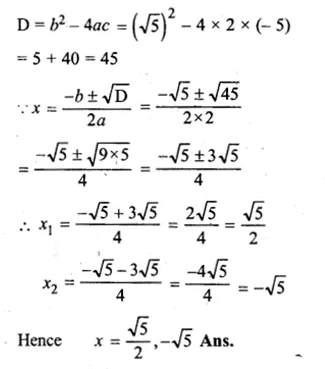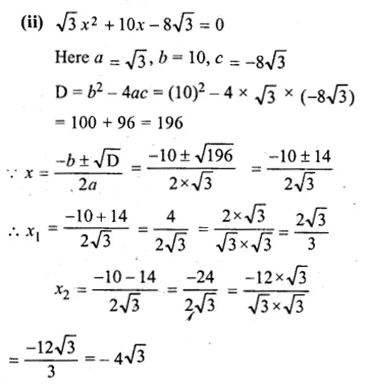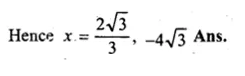Question 5.
(i) $$\frac { x-2 }{ x+2 } +\frac { x+2 }{ x-2 } =4$$
(ii) $$\frac { x+1 }{ x+3 } =\frac { 3x+2 }{ 2x+3 }$$
Solution:
(i) $$\frac { x-2 }{ x+2 } +\frac { x+2 }{ x-2 } =4$$
⇒ $$\frac { { (x-2) }^{ 2 }+{ (x+2) }^{ 2 } }{ (x+2)(x-2) } =4$$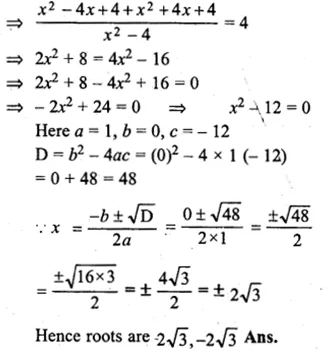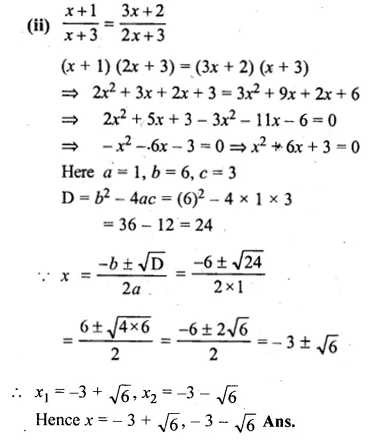Question 6.
(i) a (x² + 1) = (a² + 1) x , a ≠ 0
(ii) 4x² – 4ax + (a² – b²) = 0
Solution:
(i) a (x² + 1) = (a² + 1) x
ax² – (a² + 1)x + a = 0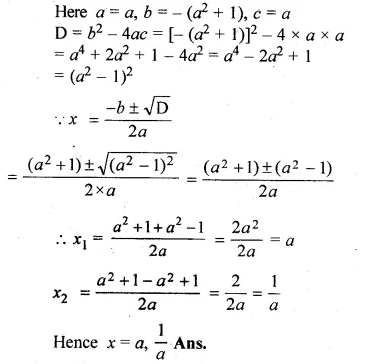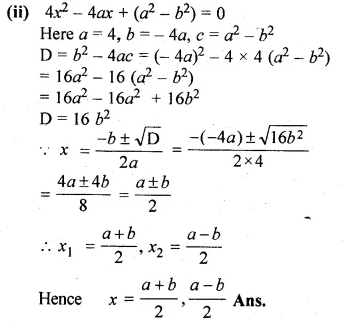Question 7.
(i)$$x-\frac { 1 }{ x } =3,x\neq 0$$
(ii)$$\frac { 1 }{ x } +\frac { 1 }{ x-2 } =3,x\neq 0,2$$
Solution:
(i)$$x-\frac { 1 }{ x } =3$$
x² – 1 = 3x
⇒ x² – 3x – 1 = 0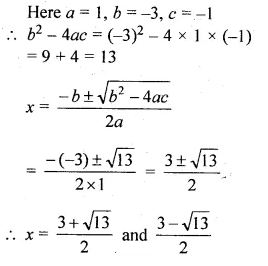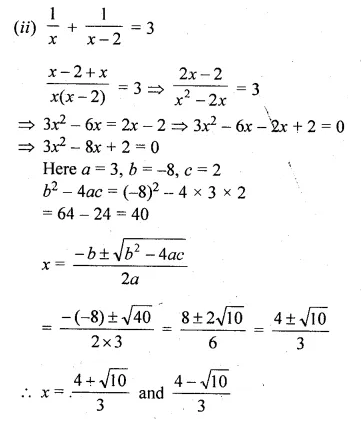Question 8.
$$\frac { 1 }{ x-2 } +\frac { 1 }{ x-3 } +\frac { 1 }{ x-4 } =0$$
Solution:
$$\frac { 1 }{ x-2 } +\frac { 1 }{ x-3 } +\frac { 1 }{ x-4 } =0$$
⇒ $$\frac { 1 }{ x-2 } +\frac { 1 }{ x-3 } =-\frac { 1 }{ x-4 }$$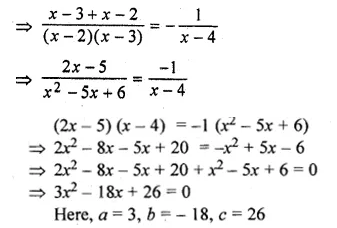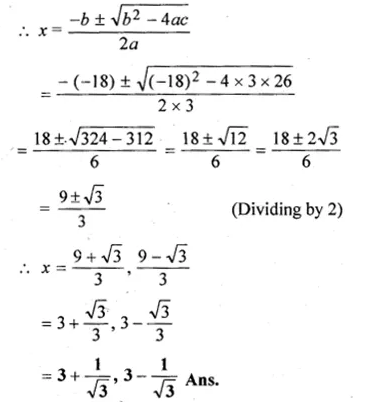Question 9.
Solve for $$x:2\left( \frac { 2x-1 }{ x+3 } \right) -3\left( \frac { x+3 }{ 2x-1 } \right) =5,x\neq -3,\frac { 1 }{ 2 }$$
Solution:
$$x:2\left( \frac { 2x-1 }{ x+3 } \right) -3\left( \frac { x+3 }{ 2x-1 } \right) =5$$
Let $$\frac { 2x-1 }{ x+3 } =y$$ then $$\frac { x+3 }{ 2x-1 } =\frac { 1 }{ y }$$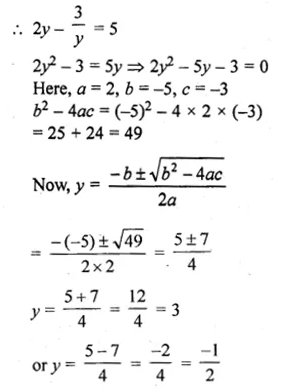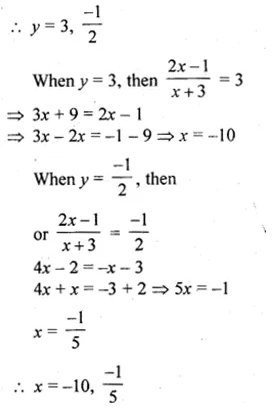Question 10.
Solve the following equation by using quadratic equations for x and give your
(i) x² – 5x – 10 = 0
(ii) 5x(x + 2) = 3
Solution:
(i) x² – 5x – 10 = 0
On comparing with, ax² + bx + c = 0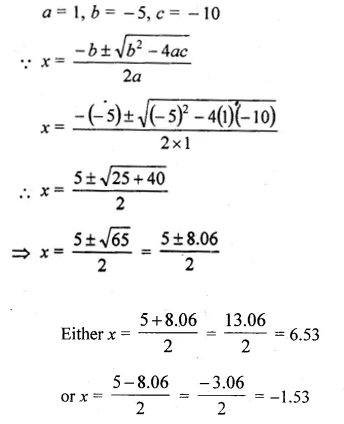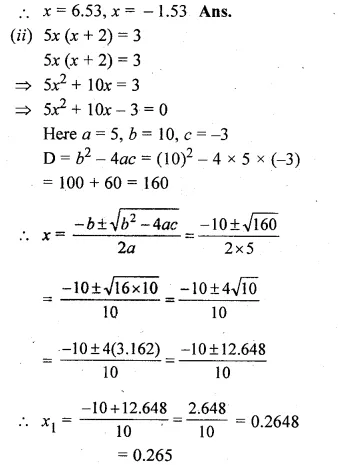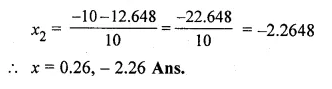Question 11.
Solve the following equations by using quadratic formula and give your answer correct to 2 decimal places :
(i) 4x² – 5x – 3 = 0
(ii) 2x – $$\\ \frac { 1 }{ x }$$ = 1
Solution:
(i) Given equation 4x² – 5x – 3 = 0
Comparing with ax² + bx + c = 0, we have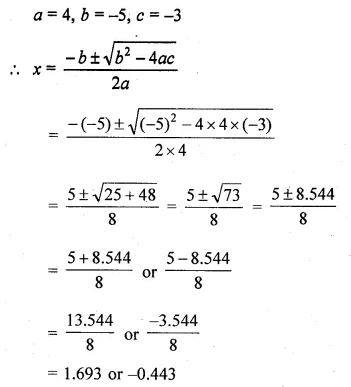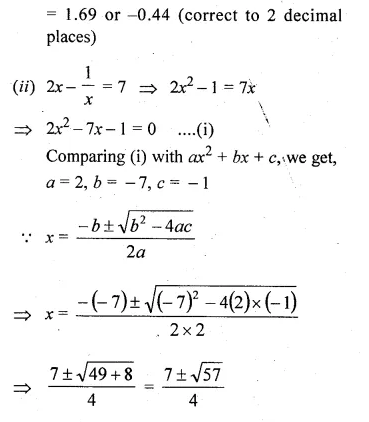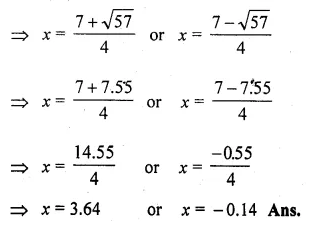Question 12.
Solve the following equation: $$x-\frac { 18 }{ x } =6$$. Give your answer correct to two x significant figures. (2011)
Solution:
$$x-\frac { 18 }{ x } =6$$
⇒ x² – 6x – 18 = 0
a = 1, b = -6, c = -18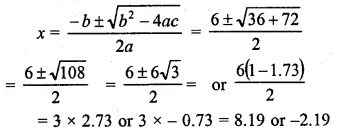Question 13.
Solve the equation 5x² – 3x – 4 = 0 and give your answer correct to 3 significant figures:
Solution:
We have 5x² – 3x – 4 = 0
Here a = 5, b = – 3, c = – 4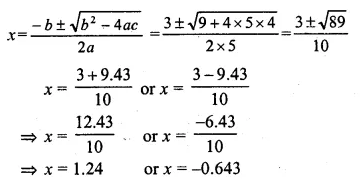Hope given ML Aggarwal Class 10 Solutions for ICSE Maths Chapter 5 Quadratic Equations in One Variable Ex 5.3 are helpful to complete your math homework.

If you have any doubts, please comment below. Learn Insta try to provide online math tutoring for you.